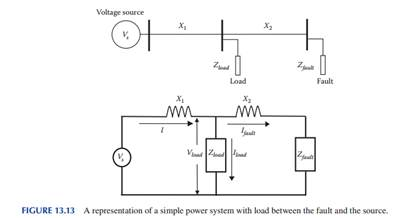### Compute the voltage sag at the load side

Assignment Help Electrical Engineering
##### Reference no: EM131208525

For the system in Figure 13.13, the source voltage is 50 kV (phase value), the feeder reactive reactance X1 is 10Ω and X2 is 5Ω. The load impedance is 3∠20° kΩ. A temporary fault occurs at the location in the figure. The fault impedance is 200∠80° Ω. Compute the voltage sag at the load side. Assume the end of the feeder was unloaded before the fault.#### Find the junction temperature rise above the room temp

If the breakdown voltage increases with temperature at a rate of 40 mV/"C, find the break-down voltage of the diode at room temperature, assuming that the space-charge resis

#### Derive the expression of the output voltage

SEE720 Assignment 1. Bridge circuits are very important in instumentation applications. They often form part of transucer circuits. Their main utility lies in the fact that

#### What is the standard deviation

A 5-mV signal is measured with a meter 10 times, resulting in the following sequence of readings: 7 mV, 6 mV, 9 mV, 8 mV, 4 mV, 7 mV, 5 mV, 7 mV, 8 mV, 11 mV. What is the me

#### Construct an incompletely specified table for the circuit

The circuit generates an output of 1 (0) when the third bit is received if the 3 bits have even (odd) parity; the circuit output is a don't-care for the first 2 bits. Constr

#### Explain telephone channels and pcm analysis

Telephone channels and PCM analysis, The analog to digital converter (ADC) used in a telephone system outputs 8 bit words. The digital words are transmitted as a serial stre

#### What is rms voltage of source in a single loop series

3) What is the RMS voltage of the source in a single loop series circuit if Irms = 1A-30° and Ztotal = 100 65° 4) What is the power dissipated by an electric heater with a res

#### Find the canonical pos of thefunction f using minterms

Consider the function f(x1,x2,x3,x4)= (x1 + x2 + ~x3 + x4)(~x1+ x4) a. Find the canonical POS of thefunction F using minterms. b. Derive the simplest POS expressionof F using

#### Which has the best time predictability for the output

Compare these three solutions (sequential, Gray, and one-hot). Which requires the fewest DFFs? Which requires the least combinational logic? Which has the best time predicta

### Write a Review